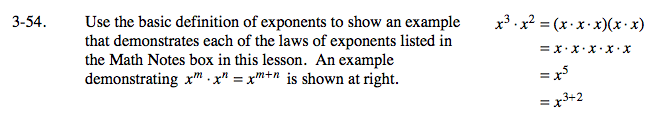### Home > AC > Chapter 14 > Lesson 14.3.1.4 > Problem3-54

3-54.

Use the basic definition of exponents to show an example that demonstrates each of the laws of exponents listed in the Math Notes box in this lesson. An example demonstrating xm · xn = xm + n is shown below. Homework Help ✎ x3 · x2 = (x · x · x)(x · x)
= x · x · x · x · x
= x5
= x3+2Look at the given example. Create your own similar examples for each of the other laws of exponents from the Math Notes box.